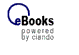Schnellsuche zur Expertensuche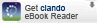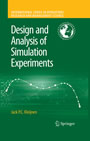Design and Analysis of Simulation Experiments
von: Jack P. C. Kleijnen
Springer-Verlag, 2007
ISBN: 9780387718132

 Format:  PDF geeignet für: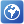Typ: B (paralleler Zugriff)InhaltsverzeichnisPreface 7 Contents 10 Introduction 13 What is simulation? 13 What is DASE? 19 DASE symbols and terms 22 Solutions for exercises 24 Low-order polynomial regression metamodels and their designs: basics 26 Introduction 27 Linear regression analysis: basics 30 Linear regression analysis: first-order polynomials 38 First-order polynomial with a single factor 38 First-order polynomial with several factors 39 Designs for first-order polynomials: resolution-III 47 2k-p designs of resolution-III 47 Plackett-Burman designs of resolution-III 50 Regression analysis: factor interactions 51 Designs allowing two-factor interactions: resolution-IV 53 Designs for two-factor interactions: resolution-V 57 Regression analysis: second-order polynomials 60 Designs for second-degree polynomials: Central Composite Designs (CCDs) 61 Optimal designs and other designs 62 Validation of metamodels 65 Coefficients of determination and correlation coefficients 65 Cross-validation 68 More simulation applications 74 Conclusions 77 Appendix: coding of nominal factors 77 Solutions for exercises 80 Classic assumptions revisited 83 Introduction 83 Multivariate simulation output 84 Designs for multivariate simulation output 87 Nonnormal simulation output 88 Realistic normality assumption? 88 Testing the normality assumption 89 Transformations of simulation I/O data, jackknifing, and bootstrapping 90 Heterogeneous simulation output variances 97 Realistic constant variance assumption? 97 Testing for constant variances 98 Variance stabilizing transformations 99 LS estimators in case of heterogeneous variances 99 Designs in case of heterogeneous variances 102 Common random numbers (CRN) 103 Realistic CRN assumption? 104 Alternative analysis methods 104 Designs in case of CRN 106 Nonvalid low-order polynomial metamodel 107 Testing the validity of the metamodel 107 Transformations of independent and dependent regression variables 108 Adding high-order terms to a low-order polynomial metamodel 108 Nonlinear metamodels 109 Conclusions 109 Solutions for exercises 110 Simulation optimization 111 Introduction 111 RSM: classic variant 115 Generalized RSM: multiple outputs and constraints 120 Testing an estimated optimum: KKT conditions 126 Risk analysis 133 Latin Hypercube Sampling (LHS) 136 Robust optimization: Taguchian approach 140 Case study: Ericsson's supply chain 145 Conclusions 147 Solutions for exercises 148 Kriging metamodels 149 Introduction 149 Kriging basics 150 Kriging: new results 157 Designs for Kriging 159 Predictor variance in random simulation 161 Predictor variance in deterministic simulation 162 Related designs 164 Conclusions 165 Solutions for exercises 166 Screening designs 167 Introduction 167 Sequential Bifurcation 170 Outline of simplest SB 170 Mathematical details of simplest SB 175 Case study: Ericsson's supply chain 177 SB with two-factor interactions 179 Conclusions 181 Solutions for exercises 182 Epilogue 183 References 185 Index 221nach oben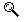Mehr zum Inhalt
 Kapitelübersicht Kurzinformation Inhaltsverzeichnis Leseprobe Blick ins BuchNavigation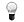Info
© 2008-2019 ciando GmbH | Impressum | Kontakt | F.A.Q. | Datenschutz